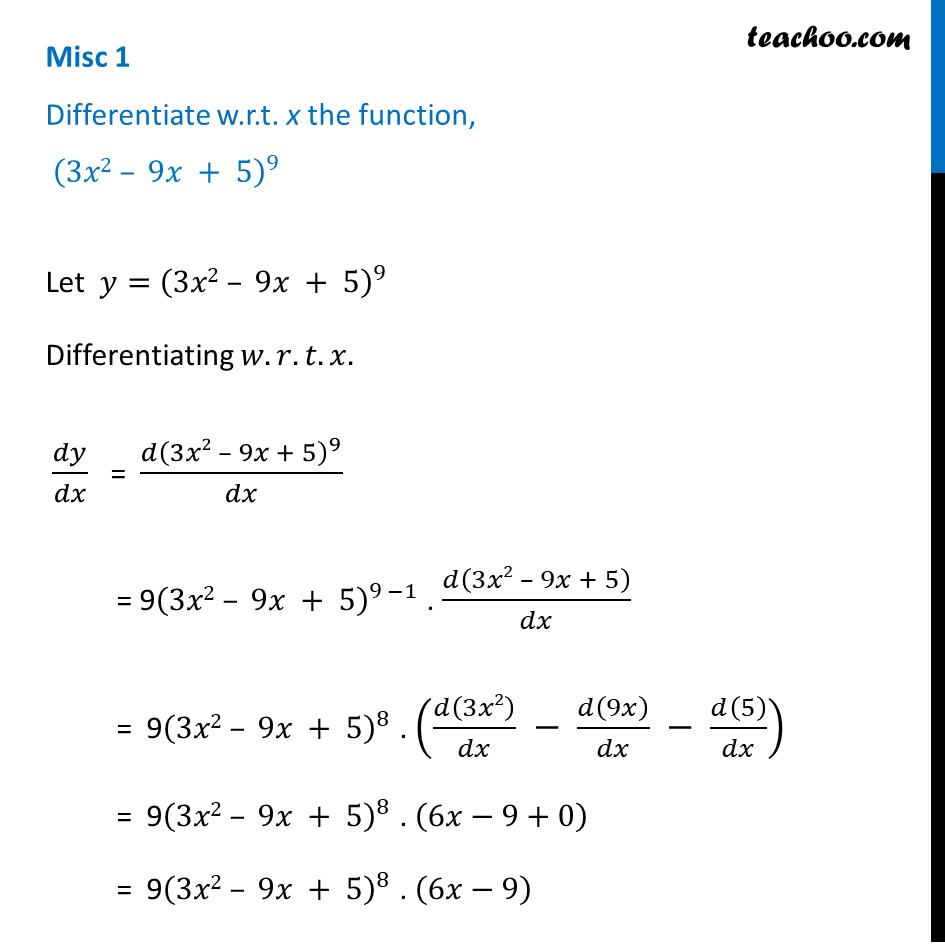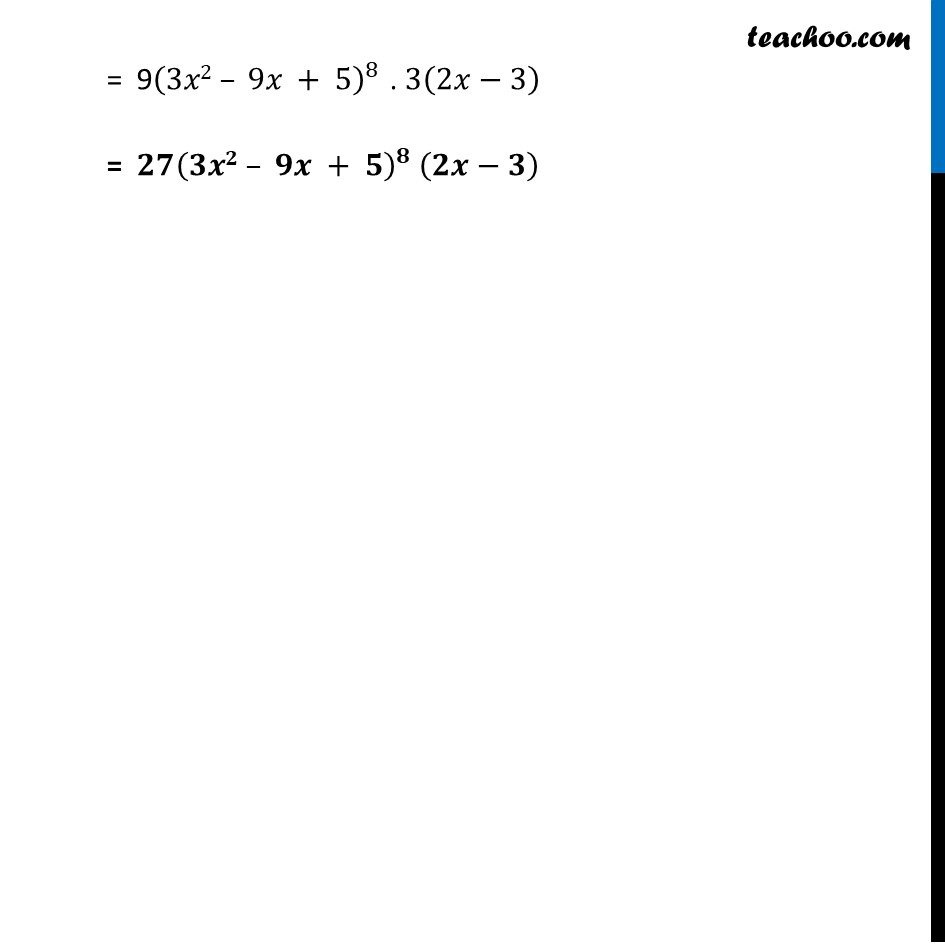Miscellaneous

Chapter 5 Class 12 Continuity and Differentiability
Serial order wiseLearn in your speed, with individual attention - Teachoo Maths 1-on-1 Class

### Transcript

Misc 1 Differentiate w.r.t. x the function, (3𝑥2 – 9𝑥 + 5)^9Let 𝑦=(3𝑥2 – 9𝑥 + 5)^9 Differentiating 𝑤.𝑟.𝑡.𝑥. 𝑑𝑦/𝑑𝑥 = (𝑑(3𝑥2 – 9𝑥 + 5)^9)/𝑑𝑥 = 9(3𝑥2 – 9𝑥 + 5)^(9 −1) . 𝑑(3𝑥2 – 9𝑥 + 5)/𝑑𝑥 = 9(3𝑥2 – 9𝑥 + 5)^8 . (𝑑(3𝑥2)/𝑑𝑥 − 𝑑(9𝑥)/𝑑𝑥 − 𝑑(5)/𝑑𝑥) = 9(3𝑥2 – 9𝑥 + 5)^8 . (6𝑥−9+0) = 9(3𝑥2 – 9𝑥 + 5)^8 . (6𝑥−9) = 9(3𝑥2 – 9𝑥 + 5)^8 . 3(2𝑥−3) = 𝟐𝟕(𝟑𝒙𝟐 – 𝟗𝒙 + 𝟓)^𝟖 (𝟐𝒙−𝟑)